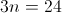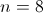## Example Questions

### Example Question #11 : Algebraic Fractions

A school's tornado shelter has enough food to last 20 children for 6 days. If 24 children ended up taking shelter together, for how many fewer days will the food last?

1

8

2

6

4

1

Explanation:

Because the number of days goes down as the number of children goes up, this problem type is inverse variation. We can solve this problem by the following steps:

20*6=24*x

120=24x

x=120/24

x=5

In this equation, x represents the total number of days that can be weathered by 24 students. This is down from the 6 days that 20 students could take shelter together. So the difference is 1 day less.

### Example Question #1 : How To Find Inverse Variation

Find the inverse equation of: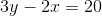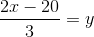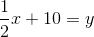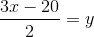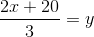Explanation:

To solve for an inverse, we switch x and y and solve for y. Doing so yields:### Example Question #7 : Algebraic Fractions

Find the inverse equation of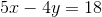.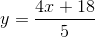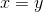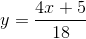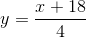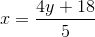Explanation:1. Switch theandvariables in the above equation.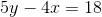2. Solve for: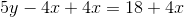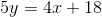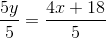### Example Question #1 : Algebraic FractionsWhen,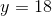.

When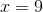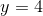.

Ifvaries inversely with, what is the value ofwhen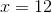?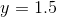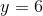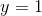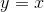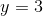Explanation:

Ifvaries inversely with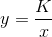.

1. Using any of the two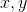combinations given, solve for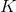:

Using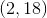: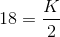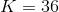2. Use your new equation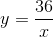and solve when: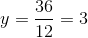### Example Question #1 : How To Find Inverse Variation

 x y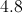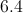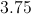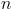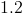Ifvaries inversely with, what is the value of?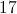Explanation:

An inverse variation is a function in the form: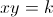or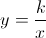, whereis not equal to 0.

Substitute each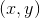in.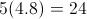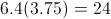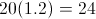Therefore, the constant of variation,, must equal 24. Ifvaries inversely as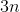must equal 24. Solve for.